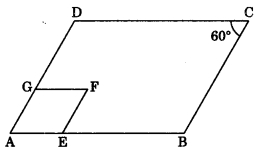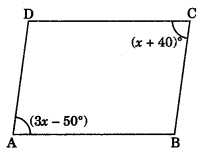CBSE Class 9 Quadrilaterals MCQ New

## CBSE Class 9 Quadrilaterals MCQ By Clarify Knowledge

CBSE Class 9 Quadrilaterals MCQ New Pattern 2022

## CBSE Class 9 Quadrilaterals MCQ Table

Question 1.
Three angles of a quadrilateral are 75°, 90°and 75°, the fourth angle is
(a) 90°
(b) 95°
(c) 105°

Question 2.
A diagonal of a rectangle is inclined to one side of the rectangle at 25°. The acute angle between the diagonals is
(a) 55°
(b) 50°
(c) 40°

Question 3.
ABCD is a rhombus such that ∠ACB = 40°, then ∠ADB is
(a) 40°
(b) 45°
(c) 50°

Question 4.
If angles A, B, C and D of a quadrilateral ABCD, taken in order, are in the ratio 3 : 7 : 6 : 4, then ABCD is a
(a) rhombus
(b) parallelogram
(c) trapezium

Question 5.
The diagonals AC and BD of a || gm ABCD intersect each other at the point O. If ∠DAC = 32° and ∠AOB = 70°, then ∠DBC is equal to
(a) 24°
(b) 86°
(c) 38°

Question 6.
ABCD is a rhombus such that ∠ABC = 40°, then ∠ADC is equal to
(a) 40°
(b) 45°
(c) 50°

Question 7.
In the following figure, ABCD and AEFG are two parallelograms. If ∠C = 60°, then ∠GFE is(a) 30°
(b) 60°
(c) 90°

Question 8.
The bisectors of any two adjacent angles of a || gm intersect at
(a) 30°
(b) 45°
(c) 60°

Question 9.
If one angle of a parallelogram is 24° less than twice the smallest angle, then the measure of the largest angle of a parallelogram is
(a) 176°
(b) 68°
(c) 112°

Question 10.
If the diagonal of a rhombus are 18 cm and 24 cm respectively, then its side is equal to
(a) 16 cm
(b) 15 cm
(c) 20 cm